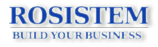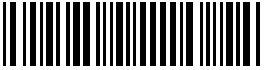First barcoding website from Romania!
 Home | About Us | Solutions | Tutorials | Barcode Education | Biometric Education | Contact Us
Barcode Education » Code 11 (USD-8)
 IntroductionCode 11 was developed in 1977 as a high-density numeric symbology. It is also known as USD-8. It is primarily used in labeling telecommunications components and equipment. Code 11 is a numeric symbology and its character set consists of 10 digital characters and the dash symbol (-). 0, 1, 2, 3, 4, 5, 6, 7, 8, 9, -. Code 11 uses one or two weighted checksum digits. Normally if the length of the message is less than 10 characters, one checksum “C” is used. Otherwise both “C” and “K” are used. Code 11 is a discrete symbology. Characters are separated by an inter-character gap which typically has the same width as the narrow element. The height of the bars must be at least 0.15 times of the symbol’s length or 0.25 inches, whichever is larger. The name derives from the way that the “C” checksum digit is calculated. For details, read further to the checksum section. Although Code 11 is discrete, it is not self-checking because a single printing defect can transpose one character into another valid character. Structure of a Code 11 barcode A typical code 11 barcode has the following structure: 1. A start character 2. Message encoded 3. The "C" check digit 4. If the length of encoded message is greater than 10, a "K" check digit is required. 5. A stop character Check Digit Calculation To calculate the optional checksum digit, follow the following steps. 1. Start with the right-most digit in the message; assign the weight starting with 1. Move from the right to left, and increment the weight by one. A dash has a value of 10. 2. Multiply the character value by the weight and add the result together. 3. Divide the total result by 11. The remainder is the “C” checksum digit. 4. If the length of the message is greater than 10, you have to calc the “K” digit as well. 5. Start with the right most digit in the message (Now it should be “C” digit). Repeat the step 1. This time divide the result by 9. The remainder becomes the “K” checksum digit.